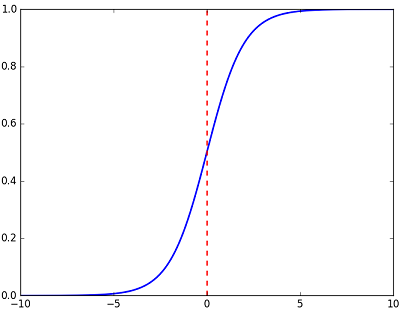# 相对熵（KL散度）

$H ( p ) = H ( X ) = \mathrm { E } _ { x \sim p ( x ) } [ - \log p ( x ) ] = -\sum_{i=1}^n p ( x )\log p ( x )$

$H ( p ) = H ( X ) = \mathrm { E } _ { x \sim p ( x ) } [ - \log p ( x ) ] = - \int p ( x ) \log p ( x ) d x$

$D _ { K L } ( p \| q ) = \sum _ { i = 1 } ^ { N } \left[ p \left( x _ { i } \right)\log p \left( x _ { i } \right) - p \left( x _ { i } \right)\log q \left( x _ { i } \right)\right]$

$D ( P \| Q ) = 0.1 \times \log \left( \frac { 0.1 } { 0.4 } \right) + 0.4 \times \log \left( \frac { 0.4 } { 0.2 } \right) + 0.5 \times \log \left( \frac { 0.5 } { 0.4 } \right)=0.250$

$D ( Q \| P ) = 0.4 \times \log \left( \frac { 0.4 } { 0.1 } \right) + 0.2 \times \log \left( \frac { 0.2 } { 0.4 } \right) + 0.4 \times \log \left( \frac { 0.4 } { 0.5 } \right)=0.327$$要证：D _ { K L } ( p \| q ) = \sum _ { i = 1 } ^ { N } \left[ p \left( x _ { i } \right)\log p \left( x _ { i } \right) - p \left( x _ { i } \right)\log q \left( x _ { i } \right)\right]\geq0$

$即证\sum _ { i = 1 } ^ { N } p (x_ { i }) \log \ \frac { q (x_ { i }) } { p (x_ { i }) } \leq0$

$又\ln ( x ) \leq x - 1，当且仅当x=1时等号成立$

$故\sum _ { i = 1 } ^ { N } p (x_ { i }) \log \ \frac { q (x_ { i }) } { p (x_ { i }) } \leq\sum _ { i = 1 } ^ { N } p (x_ { i }) (\frac { q (x_ { i }) } { p (x_ { i }) }-1)=\sum _ { i = 1 } ^ { N } [p (x_ { i })-q (x_ { i })]=0$$上面式子中\leq的等于号只在p (x_ { i })=q (x_ { i })时成立。$

### 手机扫码访问

•